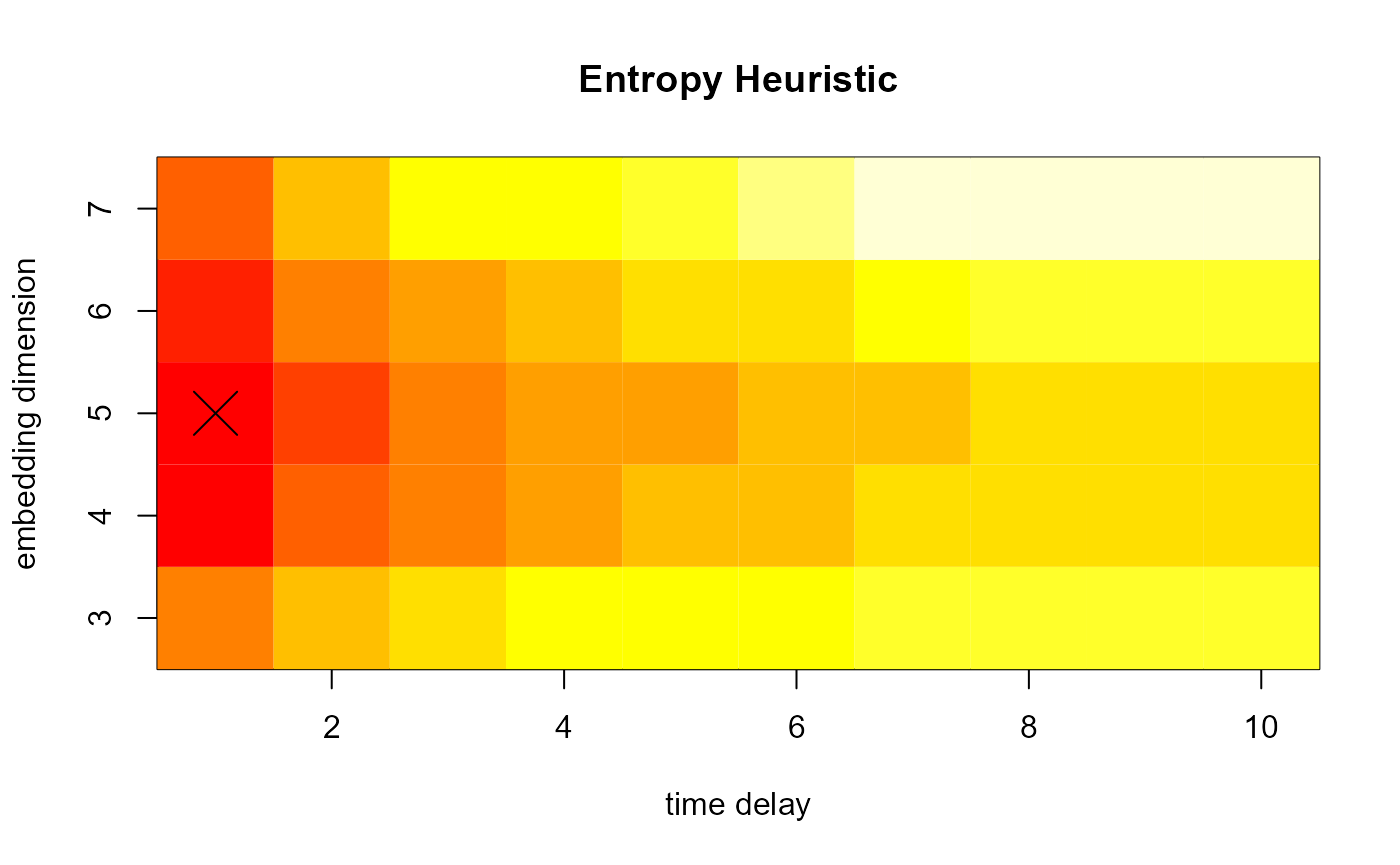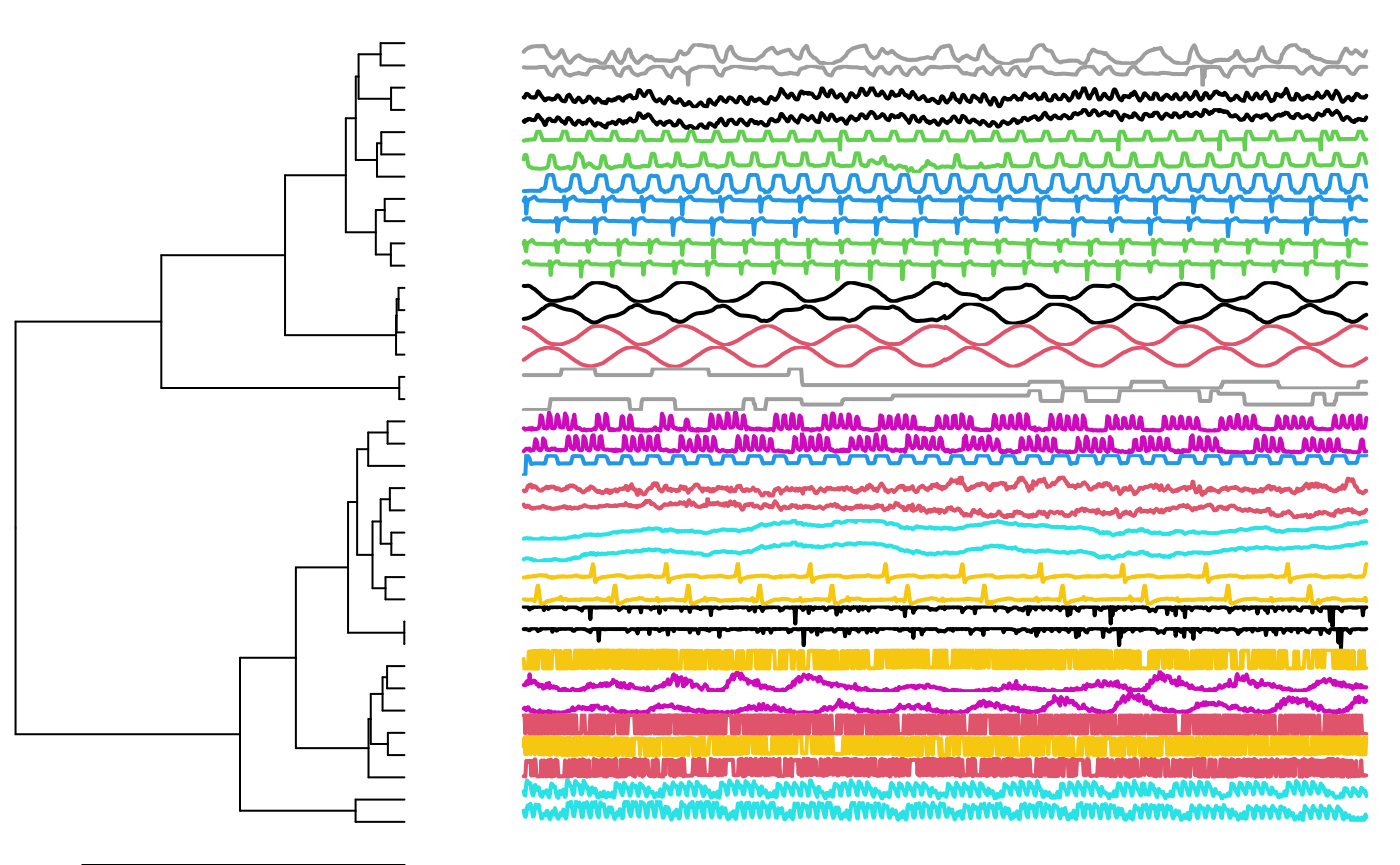This is a demonstration of how to use the pdc package to cluster time series with a data set of 18 pairs of time series. The pairs should be similar to each other but dissimilar to members of other pairs.

First, we load the paired time series data set provided by the TSclust package.

library("TSclust")
data("paired.tseries", package = "TSclust")

Plot two examples from the data set:

plot(paired.tseries[,12], main=names(colnames(paired.tseries)), ylab="")plot(paired.tseries[,2], main=names(colnames(paired.tseries)), ylab="")Run the heuristic to determine the embedding dimension and plot the result:

mine <- entropyHeuristic(paired.tseries,t.max = 10)
plot(mine)Run the clustering algorithm with the heuristic embedding dimension and give pairs of time series the same color:

clust <- pdclust(paired.tseries, m = mine$m, t = mine$t)

truth <- rep(1:18, each=2)
plot(clust, cols=truth)